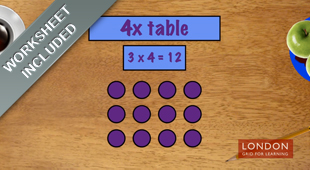# Hillcross Primary School

In this section...

# Multiplication and Division

Year 1

Multiplication & Division ProblemsYear 2

Multiplication Tables        The Commutative Law       Solving Problems
Year 3
Multiplication tablesYear 4

Mental multiplication   Multiplication tables    Multiplication

Distrubitive lawYear 5
Prime factors                     Formal Multiplication   Division with Remainders
Square & Cube Numbers       The Equals Sign              Solving problems
Year 6

Multiplying Large Numbers   Division & Remainders             Common Factors

Common Multiples                        Prime Numbers             The Order of Operations